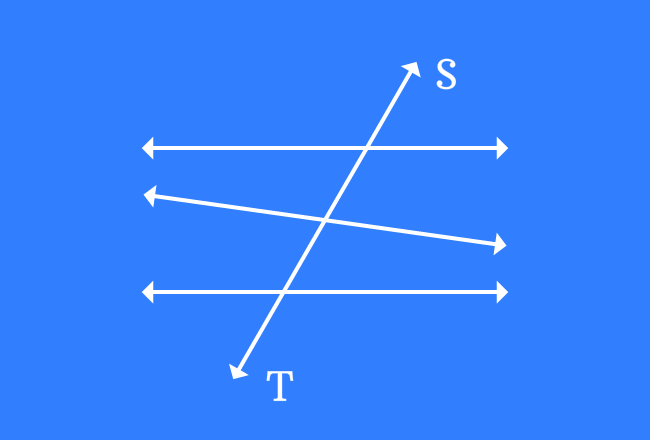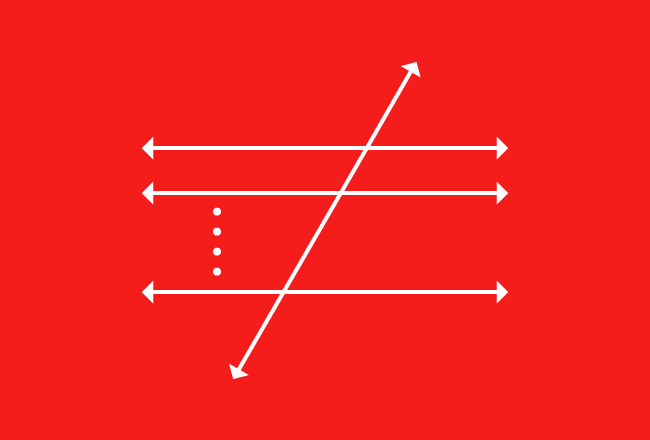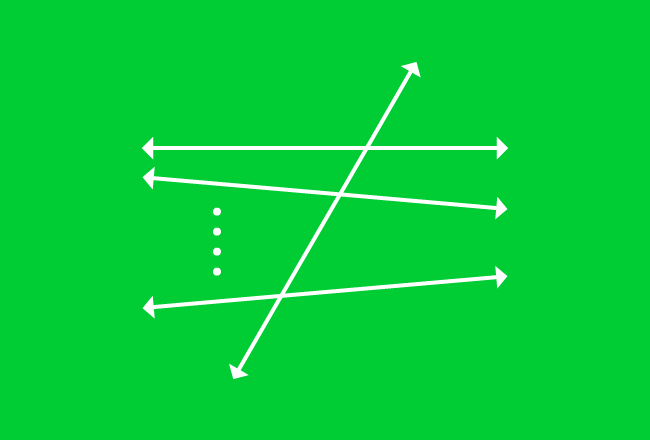# Transversal

A straight line that intersects either two or more lines is called a transversal line, or simply called as a transversal.

## IntroductionA transversal is a straight line. It intersects two or more lines and they can be either parallel or non-parallel or both types of lines.

Look at the picture. In this example, there are two parallel lines and a non-parallel line but a straight line $\overleftrightarrow{TS}$ passes through all of them by cutting three lines at different points.

The straight line $\overleftrightarrow{TS}$ is called a transversal and also called as a transversal line.

### Case study

There are two possible cases of forming transversals in geometry. So, it is very important to study them for understanding the properties of the transversal lines.

1

#### Transversal of Parallel LinesA line that intersects two or more parallel lines is called transversal of parallel lines.

2

#### Transversal of Nonparallel LinesA line that intersects two or more nonparallel lines is called transversal of non-parallel lines.

A best free mathematics education website that helps students, teachers and researchers.

###### Maths Topics

Learn each topic of the mathematics easily with understandable proofs and visual animation graphics.

###### Maths Problems

A math help place with list of solved problems with answers and worksheets on every concept for your practice.

Learn solutions

###### Subscribe us

You can get the latest updates from us by following to our official page of Math Doubts in one of your favourite social media sites.